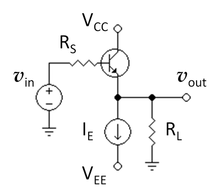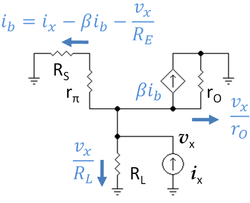# Common collector

Common collectorFigure 1: Basic NPN common-collector circuit (neglecting biasing details).

In electronics, a common-collector amplifier (also known as an emitter follower or BJT voltage follower) is one of three basic single-stage bipolar junction transistor (BJT) amplifier topologies, typically used as a voltage buffer. In this circuit the base terminal of the transistor serves as the input, the emitter is the output, and the collector is common to both (for example, it may be tied to ground reference or a power supply rail), hence its name. The analogous field-effect transistor circuit is the common-drain amplifier.

## Basic circuitFigure 2: A negative feedback amplifier

The circuit can be explained by viewing the transistor as being under the control of negative feedback. From this viewpoint, a common-collector stage (Fig. 1) is an amplifier with full series negative feedback. In this configuration (Fig. 2 with β = 1), the entire output voltage VOUT is placed contrary and in series with the input voltage VIN. Thus the two voltages are subtracted according to KVL (the subtractor from the function block diagram is implemented just by the input loop) and their difference Vdiff = VIN - VOUT is applied to the base-emitter junction. The transistor monitors continuously Vdiff and adjusts its emitter voltage almost equal (less VBEO) to the input voltage by passing the according collector current through the emitter resistor RE. As a result, the output voltage follows the input voltage variations from VBEO up to V+; hence the name, emitter follower. Intuitively, this behavior can be also understood by realizing that the base-emitter voltage in the bipolar transistor is very insensitive to bias changes, so any change in base voltage is transmitted (to good approximation) directly to the emitter. It depends slightly on various disturbances (transistor tolerances, temperature variations, load resistance, collector resistor if it is added, etc.) since the transistor reacts to these disturbances and restores the equilibrium. It never saturates even if the input voltage reaches the positive rail.

The common collector circuit can be shown mathematically to have a voltage gain of almost unity:${A_\mathrm{v}} = {v_\mathrm{out} \over v_\mathrm{in}} \approx 1$Figure 3: PNP version of the emitter follower circuit, all polarities are reversed.

A small voltage change on the input terminal will be replicated at the output (depending slightly on the transistor's gain and the value of the load resistance; see gain formula below). This circuit is useful because it has a large input impedance, so it will not load down the previous circuit:$r_\mathrm{in} \approx \beta_0 R_\mathrm{E}$

and a small output impedance, so it can drive low-resistance loads:$r_\mathrm{out} \approx {R_\mathrm{E}} \| {R_\mathrm{source} \over \beta_0}$

Typically, the emitter resistor is significantly larger and can be removed from the equation:$r_\mathrm{out} \approx {R_\mathrm{source} \over \beta_0}$

## ApplicationsFigure 4: NPN voltage follower with current source biasing suitable for integrated circuits

The low output impedance allows a source with a large output impedance to drive a small load impedance; it functions as a voltage buffer. In other words, the circuit has current gain (which depends largely on the hFE of the transistor) instead of voltage gain. A small change to the input current results in much larger change in the output current supplied to the output load.

One aspect of buffer action is transformation of impedances. For example, the Thévenin resistance of a combination of a voltage follower driven by a voltage source with high Thevenin resistance is reduced to only the output resistance of the voltage follower, a small resistance. That resistance reduction makes the combination a more ideal voltage source. Conversely, a voltage follower inserted between a small load resistance and a driving stage presents a large load to the driving stage, an advantage in coupling a voltage signal to a small load.

This configuration is commonly used in the output stages of class-B and class-AB amplifier — the base circuit is modified to operate the transistor in class-B or AB mode. In class-A mode, sometimes an active current source is used instead of RE (Fig. 4) to improve linearity and/or efficiency.

## Characteristics

At low frequencies and using a simplified hybrid-pi model, the following small-signal characteristics can be derived. (Parameter β = gmrπ and the parallel lines indicate components in parallel.)

Definition Expression Approximate expression Conditions
Current gain${A_\mathrm{i}} = {i_\mathrm{out} \over i_\mathrm{in}}$$\beta_0 + 1 \$$\approx \beta_0$$\beta_0 \gg 1$
Voltage gain${A_\mathrm{v}} = {v_\mathrm{out} \over v_\mathrm{in}}$${g_m R_\mathrm{E} \over g_m R_\mathrm{E} + 1}$$\approx 1$$g_m R_\mathrm{E} \gg 1$
Input resistance$r_\mathrm{in} = \frac{v_{in}}{i_{in}}$$r_\pi + (\beta_0 + 1) R_\mathrm{E}\$$\approx \beta_0 R_\mathrm{E}$$(g_m R_\mathrm{E} \gg 1) \wedge (\beta_0 \gg 1)$
Output resistance$r_\mathrm{out} = \frac{v_{out}}{i_{out}}$$R_\mathrm{E} || \left( {r_\pi + R_\mathrm{source} \over \beta_0 + 1} \right)$$\approx {1 \over g_m} + {R_\mathrm{source} \over \beta_0}$$(\beta_0 \gg 1) \wedge (r_\mathrm{in} \gg R_\mathrm{source})$

Where$R_\mathrm{source} \$ is the Thévenin equivalent source resistance.

### DerivationsFigure 5: Small-signal circuit corresponding to Figure 3 using the hybrid-pi model for the bipolar transistor at frequencies low enough to allow neglect of bipolar device capacitancesFigure 6: Low-frequency small-signal circuit for bipolar voltage follower with test current at output for finding output resistance. Resistor RE = RL | | rO.

Figure 5 shows a low-frequency hybrid-pi model for the circuit of Figure 3. Using Ohm's law various currents have been determined and these results are shown on the diagram. Applying Kirchhoff's current law at the emitter one finds:$(\beta+1)\frac{v_{in}-v_{out}}{R_S+r_{\pi}} = v_{out}\left(\frac{1}{R_L} + \frac{1}{r_O}\right) \ .$

Define the following resistance values:$\frac{1}{R_E} = \frac{1}{R_L} + \frac{1}{r_O}$$R=\frac{R_S+r_{\pi}}{\beta+1} \ .$

Then collecting terms the voltage gain is found as:$A_v = \frac{v_{out}}{v_{in}} = \frac{1}{1+\frac{R}{R_E}} \ .$

From this result the gain approaches unity (as expected for a buffer amplifier) if the resistance ratio in the denominator is small. This ratio decreases with larger values of current gain β and with larger vales of RE. The input resistance is found as:$R_{in} = \frac{v_{in}}{i_b} = \frac{R_S+r_{\pi}}{1-A_v} \$$=\left(R_S+r_{\pi}\right)\left(1+\frac{R_E}{R}\right ) \$$= R_S+r_{\pi} +(\beta+1)R_E \ .$

The transistor output resistance rO ordinarily is large compared to the load RL and therefore RL dominates RE. From this result, the input resistance of the amplifier is much larger than the output load resistance RL for large current gain β. That is, placing the amplifier between the load and the source presents a smaller (high-resistive) load to the source than direct coupling to RL, which results in less signal attenuation in the source impedance RS as a consequence of voltage division.

Figure 6 shows the small-signal circuit of Figure 5 with the input short-circuited and a test current placed at its output. The output resistance is found using this circuit as:$R_{out} = \frac{v_x}{i_x} \ .$

Using Ohm's law, various currents have been found, as indicated on the diagram. Collecting the terms for the base current, the base current is found as:$(\beta+1)i_b = i_x-\frac{v_x}{R_E} \ ,$

where RE is defined above. Using this value for base current, Ohm's law provides vx as:$v_x = i_b \left( R_S+r_{\pi} \right) \ .$

Substituting for the base current, and collecting terms,$R_{out} = \frac{v_x}{i_x} = R||R_E \ ,$

where || denotes a parallel connection and R is defined above. Because R generally is a small resistance when the current gain β is large, R dominates the output impedance which therefore also is small. A small output impedance means the series combination of the original voltage source and the voltage follower presents a Thévenin voltage source with a lower Thévenin resistance at its output node; that is, the combination of voltage source with voltage follower makes a more ideal voltage source than the original one.

Wikimedia Foundation. 2010.

### См. также в других словарях:

• common-collector connection — bendrakolektoris tranzistoriaus jungimas statusas T sritis automatika atitikmenys: angl. common collector connection vok. Kollektorgrundschaltung, f; Kollektorschaltung, f rus. включение транзистора по схеме с общим коллектором, n pranc.… …   Automatikos terminų žodynas

• Common emitter — Figure 1: Basic NPN common emitter circuit (neglecting biasing details). In electronics, a common emitter amplifier is one of three basic single stage bipolar junction transistor (BJT) amplifier topologies, typically used as a voltage amplifier.… …   Wikipedia

• Common base — Figure 1: Basic NPN common base circuit (neglecting biasing details). In electronics, a common base (also known as grounded base) amplifier is one of three basic single stage bipolar junction transistor (BJT) amplifier topologies, typically used… …   Wikipedia

• Common drain — In electronics, a common drain amplifier, also known as a source follower, is one of three basic single stage field effect transistor (FET) amplifier topologies, typically used as a voltage buffer. In this circuit the gate terminal of the… …   Wikipedia

• Common source — Figure 1: Basic N channel JFET common source circuit (neglecting biasing details). Figure 2: Basic N channel JFET common source circuit with source degeneration …   Wikipedia

• Common gate — Figure 1: Basic N channel common gate circuit (neglecting biasing details); current source ID represents an active load; signal is applied at node Vin and output is taken from node Vout; output can be current or voltage In electronics, a common… …   Wikipedia

• Collector (comics) — Collector Publication information Publisher Marvel Comics Fir …   Wikipedia

• Common Language Runtime — NET Framework Basisdaten Entwickler: Microsoft Aktuelle Version …   Deutsch Wikipedia

• Common Language Runtime — Pour les articles homonymes, voir CLR. Common Language Runtime (CLR) est le nom choisi par Microsoft pour le composant de machine virtuelle du framework .NET. Il s agit de l implémentation par Microsoft du standard Common Language Infrastructure… …   Wikipédia en Français

• Common coin errors — An error coin is a coin that is minted abnormally. Many different types of errors can occur during the minting process. The following are some of the most common error types. Numismatic value of error coinsMost error coins demand a premium when… …   Wikipedia

### Поделиться ссылкой на выделенное

##### Прямая ссылка:
Нажмите правой клавишей мыши и выберите «Копировать ссылку»